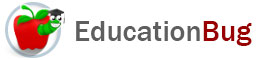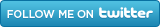PUBLIC SCHOOLS PRIVATE SCHOOLS SCHOOL DISTRICTS COLLEGES PUBLIC LIBRARIES JOBS BLOG RESOURCES
Career Education
Education Choices
Education Costs
Education History and General Information
Education Issues
Education Resources
Family Involvement in Education
Students w Special Needs
Subject Information and Homework Help
Teaching and Learning
Testing
By Types of Schools
By Specific Audiences
GeneralArea

Read this article to learn more about what area is, how to find area, and how to express the results once you have found the area. Get some basic formulas for finding area of triangles, circles, rectangles, squares, and parallelograms.

In the real estate market, in interior decorating, in planning a garden, any time a reference is made to square feet, square yards, square meters, square miles, square kilometers, acres (4840 square yards), and hectares (a unit equal to 10,000 square meters) whatâ€™s being discussed is area. To understand more about area, continue reading this article.

What Is Area?

Area is the size of a two-dimensional plane with a closed boundary. Surface area, on the other hand, is the sum of the area of the outside surfaces of a three-dimensional solid. In either case, it is expressed in square units, which in the United States may be U.S. Customary Units, or standard units, such as feet, yards, and miles or units from the International System of Units, also known as the metric system or SI, which includes meters and kilometers.

The Units of Area and Their Names

Letâ€™s begin by looking at the units of area. Area is found by multiplying one distance by another distance, both expressed in exactly the same units. Distance is expressed by units like feet, yards, meters, miles, and kilometers. The results are expressed in square units, which can be written with the word square, the abbreviation sq., or the superscript 2 following the unit, and the unit may be abbreviated as well, like this:

 square feet sq. feet sq. ft. feet2 or ft.2 square meters sq. meters sq. m meters2 or m2 square yards sq. yards sq. yd. yards2 or yd.2 square miles sq. miles sq. mi. miles2 or mi.2 square kilometers sq. kilometers sq km kilometers2 or km2

Note that in the SI system, not only are the same abbreviations used for singular and plural forms of the same unit, but no periods are used. On the other hand, with standard units, periods are used in some style systems, although it is similar to SI in using the same abbreviations for singular and plural units. The units called acres and hectares are only used to express the results, not to find the area and they are units of area, so do not have the indication square at any time or in any form.

The Units of Area and How They Relate

Here

 Unit Conversion New Unit ft.2 0.09290304 m2 m2 10.7639104 ft.2 yd.2 0.83612736 m2 m2 1.19599005 yd.2 mi.2 2.58998811 km2 km2 0.386102159 mi.2

Note that for many purposes, it might be appropriate to round these numbers. To read more about rounding, see the article â€œRounding Numbers.â€

Formulas for Area

The standard formulas for area can be quite useful. Here are the formulas for basic geometric shapes (for more on shapes, see the article â€œGeometric Shapesâ€).

• Triangle

A triangle is a polygon with three sides. The formula for the area of a triangle is:

A = Â½ bh

where A is the area, b is the length of the base, and h is the height of the figure. In a triangle that is not equilateral (that is, when the sides are not all the same length), one may choose any side to be the base.

• Parallelogram

A parallelogram is a polygon with four sides (a quadrilateral) and two sets of parallel sides. The formula for the area of a parallelogram is:

A = bh

where A is the area, b is the length of the base, and h is the height of the figure. You might want to take a moment to compare this to the formula for a triangle, so you see the relationship and donâ€™t confuse them.

• Rectangles and Squares

A rectangle is a quadrilateral with four right angles. Since the base of a rectangle is the length of one set of sides and the height is the length of the other set of sides, the area of a rectangle can be expressed like this:

A = ab

where A is the area, a is the length of one side, and b is the length of the other side.

For squares, which are rectangles with equal and parallel sides, the formula can be simplified to this:

A = s2

where A is the area and s is the length of one side.

• Circles

A circle is a geometric figure with a boundary formed of points that are equidistant from its center. The length from the center to any point on the perimeter is called the radius (for more about perimeter, see the article of that name). The formula for the area of a circle is

A = Ï€r2

where A is the area, Ï€ is the constant pi (3.14159), and r2 is the radius squared.